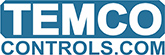# Calculating week-to-date hours

Since the DOW (day of the week) begins with zero I was simply going to take DOW * 24 to get elapsed hours for the week. But how to parse the time to get elapsed hours since midnight? I set a variable to “Time” and 16:06 shows up in the variable list as 57994.xxx
So how can I get the hours section of the current time?

1 Like

I resolved this by dividing TIME by 3600 to get the decimal number of hours elapsed since midnight

1 Like

If there’s something I can add to the documentation here let me know.

I would just add a line or two that say the TIME variable returns the number of seconds that have elapsed since midnight. Divide by 3600 to get the decimal number of hours. This is same as decimal time.

Ted